# 0 Introduction

## 0.0 Book Resources

• Artificial Intelligence A Modern Approach, 3rd Edition

## 0.1 AI

• Artificial intelligence (AI) is the branch of computer science that develops machines and software with intelligence.

## 0.2 The Turing Test

• The Turing Test was designed to provide a satisfactory operational definition of intelligence.
• 2 people & 1 machine
• an interogator: to find out which candidate is the machine or human, only by asking them questions.
• If the machine can fool the interrogator 30% of the time, the machine is considered intelligent.

# 1 Problem Formulation

## 1.1 Problem Formulation

• Problem formulation is the process of deciding what actions and states to consider, given a goal.

## 1.2 Problem Components

• Initial State: the starting state of the problem, defined in a suitable manner.

• Actions (Operators): an action or a set of actions that moves the problem from one state to another.

• Neighbourhood: ­The set of all possible states reachable from a given state by applying all legal action(s).
• Successor Function: the action(s).
• Goal Test: a test applied to a state which returns true if we have reached a state that solves the problem.

• Path Cost: how much it costs to take a particular sequence of actions.

• A solution to a problem is an action sequence that leads from the initial state to the a goal state.
• State Space: the set of all states reachable from the initial state (it forms a directed network or graph), determined by the initial state and the successor function, decides the the complexity of a problem based on its size.

## 1.3 Problem Representation - Graph

• Graph: to model the deeper structure of a problem
• Consists:
• A set V of nodes
• has a unique label for identification
• represents possible stages of a problem
• A set E of edges
• is the connection between two nodes
• represents inferences, moves in a game, or other steps in a problem solving process (operator)

## 1.4 Search Trees

• Tree: a graph that
• is connected but becomes disconnected on removing any edge
• is connected and acyclic
• has precisely one path between any two nodes• Nodes
• Root node
• Children / parent of nodes
• Leaves
• Branches
• Average branching factor: average number of branches of the nodes in the tree
• Depth
• of a node: the number of edges (branches) away from the root node
• of a tree: the depth of the deepest nodeProblem Representation

• State: nodes
• Initial State: root node
• State Space: all nodes in the tree
• Neighborhood: the set of all possible states reachable from a given state
• Number of neighborhoods: branching factor
• Operator(s): branches

How big can a search tree be?

• A branching factor b
• One goal exists at depth d
• bd different branches in the tree
• Complete Search Methods' properties
• If a goal exists then the search will always find it
• If no goal exists then the search will eventually finish and be able to say for sure that no goal exists
• Completeness: ­Is the strategy guaranteed to find a solution?
• Time Complexity: ­How long does it take to find a solution?
• Space Complexity: ­How much memory does it take to perform the search?
• Optimality: ­Does the strategy find the optimal solution where there are several solutions?

## 1.7 Search Tree Issues

• Wasteful on space
• Difficult to implement
• Not always obvious which node to expand next. We may have to search the whole tree looking for the best leaf node. This can obviously be computationally expensive.

# 2 Search Strategies - Blind Search

## 2.1 Categories

• Uninformed / Blind Search
• BFS
• DFS
• UCS
• Informed / Heuristic Search
• Greedy Search
• A* Search

### 2.2.1 Fundamental actions

• Expand: ask a node for its children
• Test: test a node to see whether it is a goal

Function General-Search(p, QUEUING-FN) returns a solution or failure.

Function General-Search(p, QUEUING-FN) returns a solution or failure.

### 2.2.4 Search Strategies

• Strategy: picking the order of node expansion
• Evaluating strategies
• Completeness: does it always find a solution if one exists?
• Time complexity: number of nodes generated/expanded
• Space complexity: maximum number of nodes in memory
• Optimality: does it always find a least-cost solution?

Time and space complexity are measured in terms of

• b: maximum branching factor of the search tree
• d: depth of the least-cost solution
• m: maximum depth of the state space (may be ∞)• Method

• Expand shallowest unexpanded node
• Expand Root Node First
• Expand all nodes at level 1 before expanding level 2
• Expand all nodes at level d before expanding nodes at level d+1
• Queuing function: adds nodes to the end of the queue
• General-Search(problem, ENQUEUE-AT-END)
• Evaluation

• Optimal: yes (only if the branch costs are the same)
• Complete: yes
• Time complexity: O(bd) i.e. the number of leaves
• Space complexity O(bd) i.e. the total number of nodes

b: the average branching factor

d: the depth of the search tree• Method
• Expand Root Node First
• Explore one branch before exploring another branch
• Queuing function: adds nodes to the front of the queue
• Function DFS(problem) returns a solution or failure
• Return General-Search(problem, ENQUEUE-AT-FRONT)
• Evaluation
• Optimal: no
• Complete: no
• Time complexity: Θ(|V| + |E|), bm
• Space complexity O(|V|), bm

### 2.2.6 Uniform Cost Search (Cheapest Fisrt Search) (VS. BFS)• Method
• Always remove the smallest cost node first
• ­Sort the queue in an increasing order, or
• ­Search the queue and remove the node of smallest cost
• Function UCS(problem) returns a solution or failure
• Return General-Search(problem, ENQUEUE-BY-COST)
• Evaluation
• Optimal: yes (even branch costs are different)
• Complete: yes
• Time complexity: O(bd) i.e. the number of leaves
• Space complexity O(bd) i.e. the total number of nodes
• VS. BFS
• UCS = BFS (when all branches have the same cost (in the worst case)
• UCS is usually better than BFS

# 3 Search Strategies - Heuristics

## 3.1 Several AI Methods

• Heuristics
• Fuzzy Logic
• Bayes reasoning
• Game Theory
• Evolutionary Computation
• Evolutionary Game Theory

## 3.2 Definition of Heuristics

• A heuristic is any approach to problem solving, learning, or discovery that employs a practical method not guaranteed to be optimal or perfect, but sufficient for the immediate goals. Where finding an optimal solution is impossible or impractical, heuristic methods can be used to speed up the process of finding a satisfactory solution.

## 3.3 Types of Solutions

• A solution to an optimization problem specifies the values of the decision variables, and therefore also the value of the objective function.
• A feasible solution satisfies all constraints.
• An optimal solution is feasible and provides the best objective function value.
• A near-optimal solution is feasible and provides a superior objective function value, but not necessarily the best.

## 3.4 Continuous & Combinatorial

• Continuous: optimization problems that has an infinite number of feasible solutions
• Continuous problem generally maximize or minimize a function of continuous variables such as min 4x + 5y where x and y are real numbers
• Combinatorial: optimization problems that has a finite number of feasible solutions
• Combinatorial problems generally maximize or minimize a function of discrete variables such as min 4x + 5y where x and y are countable items (e.g. integer only)

## 3.5 Constraints

• Hard Constraints: must be satisfied
• Soft Constraints: desirable to satisfy
• Explicit Constraints: stated in the problem
• Implicit Constraints: obvious to the problem

## 3.6 Search Basics

• Search: the term used for constructing/improving solutions to obtain the optimum or near-optimum.
• Solution: encoding (representing the solution)
• Neighborhood: nearby solutions (in the encoding or solution space)
• Move: transforming current solution to another (usually neighboring) solution
• Evaluation: the solutions’ feasibility and objective function value
• Constructive: search techniques work by constructing a solution step by step, evaluating that solution for (a) feasibility and (b) objective function
• Improvement: search techniques work by constructing a solution, moving to a neighboring solution, evaluating that solution for (a) feasibility and (b) objective function
• Properties: Search techniques may be deterministic or stochastic
• Heuristic Search (Informed Search): Add domain-specific information to select the best path along which to continue searching
• Heuristic search works by deciding which is the next best node to expand (there is no guarantee that it is the best node)
• A heuristic method is particularly used to rapidly come to asolution that is hoped to be close to the best possible answer, or 'optimal solution’

Blind Search vs. Heuristic Searches

• Blind search
• Blindly choose where to search in the search tree
• When problems get large, not practical any more
• Heuristic search
• Explore the node: more likely to lead to the goal state
• Using knowledge, so called informed search
• Heuristics: "rules of thumb", educated guesses, intuitive judgments or simply common sense.
• Greedy search, A* search

## 3.8 Heuristic Function

• h(n) estimates the “goodness” of a node n
• specifically, h(n) = estimated cost (or distance) of minimal cost path from n to a goal state
• h(n) ≥ 0 for all nodes n and h(n) = 0 implies that n is a goal node
• Use as an evaluation function f(n) = h(n), sorting nodes by increasing values of f
• Selects node to expand believed to be closest(hence“greedy”) to a goal node (i.e., select node with smallest f value)
• Pros: simple, easy to implement, run fast
• Cons: often do not provide a globally optimum solution (but a locally optimum solution)
• Not complete: A complete heuristic always reaches the goal if thereexists a route
• Time and space complexity is O(bd)
• Not admissible: An admissible heuristic never overestimates the cost of reaching the goal

https://youtu.be/3XaqEng_K5s

Greedy Search vs UCS

## 3.11 A* Algorithm

https://youtu.be/6TsL96NAZCo

• Combines the cost so far and the estimated cost to the goal f(n) = g(n) + h(n)
• g - cost from the initial state to the current state so far
• h - the cost from the current state to a goal state (underestimated)
• f - an evaluation of the state: estimated cost of the cheapest solution through n

Notes:

• To be optimal and complete, the heuristic must be admissible
• Admissible: must use a heuristic h(n) that never overestimate the cost
• Methods:
• Euclidean distance (straight line distance)
• Manhattan distance (the sum of absolute pitch)
• When h = 0, A* becomes UCS => complete & optimal*, but search undirected
• When h too large thus dominates g, A* becomes becomes Greedy => lose optimality

Informedness

• For two A* heuristics h1 and h2, if h1(n) <= h2(n) for all states n in the search space
• we say h2 dominates h1 or heuristic h2 is more informed than h1.
• Means:
• (In efficieny,) A* using h2 will never expand more nodes than using h1.
• Hence:
• it is always better to use a heuristic function with higher values
• provided it does not over-estimate
• and that the computation time for the heuristic is not too large.

# 4 Fuzzy Sets & Game Theory

## 4.1 Fuzzy Logic

### 4.1.1 What

• Fuzzy logic is a form of many-valued logic in which the truth values of variables may be any real number between 0 and 1. It is employed to handle the concept of partial truth, where the truth value may range between completely true and completely false.

### 4.1.2 Why

• Ordinary (Boolean) logic assumes things to be true or false. No grey in between.
• Ordinary maths is very exact and ignores the uncertainty
• Statistics can be used but have assumptions built in
• Fuzzy Logic is model free

## 4.2 Utility Theory

### 4.2.1 Strong AI vs Weak AI

• Strong AI: AI that matches or exceeds human intelligence
• Weak AI: machines can demonstrate intelligence, but do not necessarily have a mind, mental states or consciousness

### 4.2.2 Some Basic Concepts

• Decision Making: The act of choosing between two or more courses of action, or choosing between possible solutions to a problem.
• Rationality: the quality or state of being rational, that is, being based on or agreeable to reason.
• Utility is an abstract measure for the amount of satisfaction you receive from something (mainly in Economics). For example, one receives utility from eating food, watching movies, and etc.
• Two axiomatic assumptions on rationality:
1. In any given situation a decision-maker always chooses the action which is the best according to his/her utility or preferences.
2. Rational choice is common knowledge among all relevant decision makers.
• Rational choice: weight the advantages and disadvantages of different options (or actions), and then choose the action that maximize individual utility.

### 4.2.3 Law of Diminishing Marginal Utility (Gossen's First Law)

• Marginal Utility: the change in the utility from an increase (or decrease) in the consumption of that good or service.

• Law of Diminishing Marginal Utility: the first unit of consumption of a good or service yields more utility than the second and subsequent units, with a continuing reduction for greater amounts. Therefore, the fall in marginal utility as consumption increases is known as diminishing marginal utility.

MU1>MU2>MU3......>MUn

where MUn = Un - Un-1 means marginal utility of the nth unit.

### 4.2.4 Expected Utility Hypothesis(Theory)

• Expected Utility Hypothesis: concerning people's preferences with regard to choices that have uncertain outcomes (gambles), states that if specific axioms are satisfied, the subjective value associated with an individual's gamble is the statistical expectation of that individual's valuations of the outcomes of that gamble.

EU = ∑ pi Ui

where Ui is the utility of ith outcome, and pi is probability of ith outcome.

### 4.2.5 Decision Making under Uncertainty

• Uncertainty: the lack of certainty, a state of limited knowledge where it is impossible to exactly describe the existing state, a future outcome, or more than one possible outcome.
• Probability based methods
• Bayesian reasoning
• Expected utility theory
• Game theory
• ...
• Soft computing (non-probability) methods
• Fuzzy logic
• Interval probability theory
• Evolutionary computation
• Machine learning
• ...

### 4.2.6 Classic Game Theory

#### 4.2.6.1 Game

• Game: a situation where the participants’ payoffs depend not only on their decisions, but also on their rivals’ decisions.

• Types:
• Cooperative Game / Non-cooperative Game
• Static Game / Multi-stage Game
• Static: game is played only once (Prisoners’ dilemma)
• Multi-stage: game is played in multiple rounds (chess)
• Complete Information / Incomplete Information
• Complete info.: players know each others’ payoffs (Prisoners’ dilemma)
• Incomplete info.: other players’ payoffs are not known (Sealed auctions)
• Simultaneous Play Game / Sequential Play Game
• Simultaneous Play Game: player chose their strategies simultaneously. (Payoffs can be described by a matrix.)
• Sequential Play Game: one player (leader) plays before another player (follower). (Payoffs can be described by game tree.)
• Game Theory: the study of mathematical models of conflict and cooperation (Strategic Interactions) between intelligent rational decision-makers.

• Three elements to describe a game:

• Players: I
• Strategies: S (options each player can choose)
• Payoffs: P (how much utility each player receives for any specific outcome)

Mathematically, a game can be described by a 3-tuple G = {I, S, P}

#### 4.2.6.2 Strategies

• Strategy: Decision-maker’s choice(s) in any given situation

• Strategy Space: set of all possible actions

• Finite / Infinite

• Pure / Mixed

• Pure strategy: deterministic actions
• Mixed strategy: randomized actions (with probability)

E.g. in Rock-Paper-Scissors game

• three pure strategies
• numerous mixed strategies
• a possible one: a player plays rock, paper and scissors each with 1/3 probability

#### 4.2.6.3 Payoffs

• Payoffs: Decision-maker’s utility in any given situation

• Payoff Space: set of all possible outcomes

• Described by a Tree or Matrix

E.g. in Rock-Paper-Scissors game#### 4.2.6.4 Game Theory

• Game theory: the study of how people behave in strategic situations.
• Two classical game theory branches:
• Cooperative Game Theory: In a cooperative game, the players try to form coalitions. Within a coalition, the players make choices collectively to maximize the payoff of the coalition.
• Non-cooperative Game Theory: In a non-cooperative game, the players make their choices independently in order to maximize their own payoffs.

#### 4.2.6.5 Dominant and Dominated Strategy

• Dominant Strategy: a strategy that gives higher payoffs no matter what the opponent does.
• Dominated Strategy: a strategy is dominated if there exists another strategy that is dominant. (i.e. a strategy that gives lower payoffs no matter what the opponent does.)

E.g. in Prisoners' Dilemma:Whatever Prisoner A chooses, best choice for Prisoner B is always ‘Confess’ because -1 < 0 and -10 < -5. Confess’ is dominant strategy for B. So, in the prisoner’s dilemma. ‘Confess’ is dominant strategy for both players and ‘Keep silence’ is dominated strategy for both players.

But, in most games there are no dominant strategies for all players. We cannot use this method to predict the outcome of the game. Nash equilibrium analysis is a general tool for all games.

#### 4.2.6.6 Nash Equilibrium

• Nash Equilibrium: a solution concept of a non-cooperative game involving two or more players in which each player is assumed to know the equilibrium strategies of the other players, and no player has anything to gain by changing only his own strategy. (i.e. each player is choosing the best strategy, given the strategies chosen by the other players.)

If each player has a dominant strategy, the state that each player adopts their dominant strategies is Nash equilibrium. (e.g. ‘Confess, Confess’ in Prisoners' Dilemma)

• Nash’s Theorem: A non-cooperative game involving two or more players has at least one Nash equilibrium, possibly involving mixed strategy. (J. F. Nash, 1950)

Computing Nash equilibrium:

• Pure strategy Nash equilibrium (easy to compute for finite strategy space)
• Mixed strategy Nash equilibrium (hard to compute when the strategy space is not small)

Example of Computing Mixed Strategies Nash Equilibrium:If B plays Left, A's expected payoff is 2pU + 5(1- pU)

If B plays Right, A's expected payoff is 4pU + 2(1- pU)

If 2pU + 5(1- pU) > 4pU + 2(1- pU), then B would play only Left. But there are no Nash equilibria in which B plays only Left.

If 2pU + 5(1- pU) < 4pU + 2(1- pU), then B would play only Right. But there are no Nash equilibria in which B plays only Right.

So for there to exist a Nash equilibrium, B must be indifferent between playing Left or Right; i.e. 2pU + 5(1- pU) = 4pU + 2(1- pU) or pU=3/5

Similarly, for there to exist a Nash equilibrium, A must be indifferent between playing Up and Down; i.e. pL = 3-3pL or pL=3/4

So the game’s only Nash equilibrium has A playing the mixed strategy (3/5, 2/5) and has B playing the mixed strategy (3/4, 1/4)A's expected Nash equilibrium payoff is: 1 * 3/5 * 3/4 + 0 * 3/5 * 1/4 + 0 * 2/5 * 3/4 + 3 * 2/5 * 1/4 = 3/4

B's expected Nash equilibrium payoff is: 2 * 3/5 * 3/4 + 4 * 3/5 * 1/4 + 5 * 2/5 * 3/4 + 2 * 2/5 * 1/4 = 16/5

How many Nash equilibria?

• a finite number of players, a finite number of pure strategies => at least one Nash equilibrium
• no pure strategy Nash equilibrium => at least one mixed strategy Nash equilibrium

#### 4.2.6.7 Minimax

• Minimax: a decision rule for minimizing the possible loss for a worst case scenario.
• (to solve zero-sum games that can be described by a game tree.)
• Minimax Value: the smallest value that the other players can force the player to receive. If a player adopts the minimax strategy, the minimax value can be guaranteed no matter what strategies other players adopt.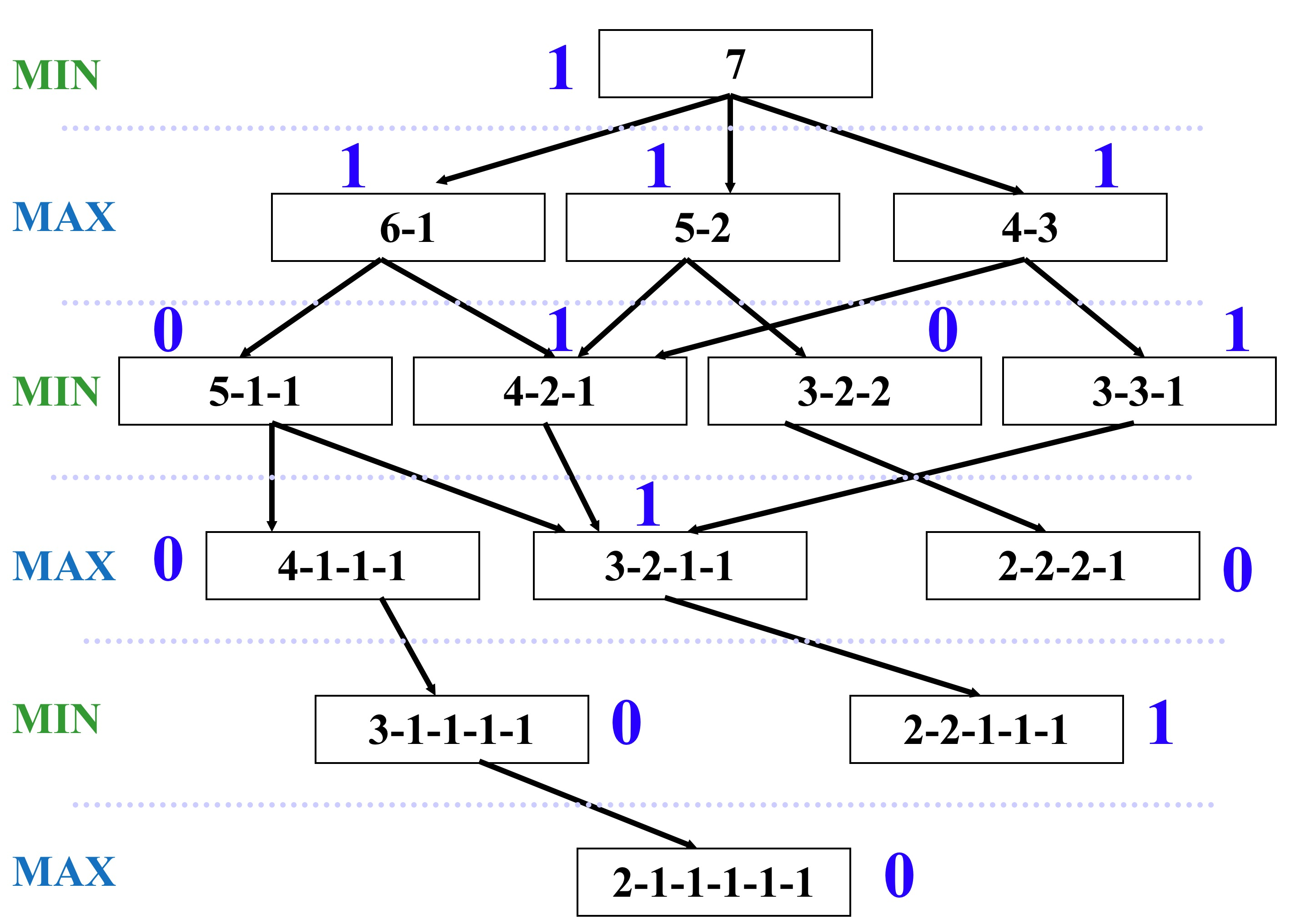https://youtu.be/zp3VMe0Jpf8

### 4.2.7 Evolutionary Game Theory (EGT)

#### 4.2.7.1 Problems with Classical Game Theory

• Assumption of rationality: not always appropriate
• Limitation of Nash equilibrium: maybe too many Nash equilibria
• Difficulty in computing Nash equilibrium: NP hard

#### 4.2.7.2 Some Basic Concepts

• Darwinian evolution is based on three fundamental principles: reproduction, mutation and selection.
• Evolutionary Game Theory (EGT) is the application of game theory to evolving populations.
• An evolutionary version of game theory does not require players to act rationally – only that they have a strategy.
• It focus on the dynamics of strategy change in the population.
• Genotype: the genetic type of a player
• Phenotype: the behavior of a genotype
• Fitness: a measure of the success of a phenotype
• Selection: successful genotypes out-reproduce unsuccessful ones
• Mutations: random creation of new genotypes
• Invasion: mutations that successfully out-compete the current genotypes and increase in number
• Evolutionarily Stable Strategy (ESS): is a strategy which, if adopted by a population in a given environment, cannot be invaded by any alternative strategy that is initially rare.

#### 4.2.7.3 Models of EGTEGT encompasses Darwinian evolution, including competition (the game), natural selection (replicator dynamics), and heredity.

Components:

• Population
• Game
• Replicator Dynamics

Phases:

1. The model (as evolution itself) deals with a Population (Pn). The population will exhibit Variation among Competing individuals. In the model this competition is represented by the Game.
2. The Game tests the strategies of the individuals under the “rules of the game”. These rules produce different payoffs – in units of Fitness (the production rate of offspring). The contesting individuals meet in pairwise contests with others, normally in a highly mixed distribution of the population. The mix of strategies in the population affects the payoff results by altering the odds that any individual may meet up in contests with various strategies. The individuals leave the game pairwise contest with a resulting fitness determined by the contest outcome, represented in a Payoff Matrix.
3. Based on this resulting fitness each member of the population then undergoes replication or culling determined by the exact mathematics of the Replicator Dynamics Process. This overall process then produces a New Generation P(n+1). Each surviving individual now has a new fitness level determined by the game result.
4. The new generation then takes the place of the previous one and the cycle repeats. The population mix may converge to an Evolutionary Stable State that cannot be invaded by any mutant strategy.

#### 4.2.7.4 Replicator Dynamics

• Replicator Equation: a deterministic monotone non-linear and non-innovative game dynamic used in evolutionary game theory.where:

• xi is the proportion of type i in the population
• x = (x1, ..., xn) is the vector of the distribution of types in the population
• fi(x) is the fitness of type i (which is dependent on the population)
• φ(x) is the average population fitness (given by the weighted average of the fitness of the n types in the population)• Evolutionarily Stable State: population's genetic composition is restored by selection after a disturbance, provided the disturbance is not too large. Such a population can be genetically monomorphic or polymorphic.

• Evolutionarily Stable Strategy (ESS): a strategy which, if adopted by a population in a given environment, cannot be invaded by any alternative strategy that is initially rare.

• ESS = Nash Equilibrium + Stability Condition

• E(S, T): the payoff for palying strategy S against stategy T
• If S is an ESS, then for all T ≠ S
• E(S, S) ≥ E(T, S) and E(S, T) > E(T, T)
• Some facts:
• ESSes and Nash equilibria often coincide.
• Every ESS corresponds to a Nash equilibrium, but some Nash equilibria are not ESSes.
• Some games have ESS, some don’t have.Example: The Prisoners Dilemma• Payoff:
• T>R>P>S, 2R>T+S
• => Defect is an ESS (and the only Nash equilibrium in this game)
• 2 genotypes in the population
• Cooperators (C-types): always cooperate
• Defectors (D-types): always defect
• (Suppose that the proportions of cooperators and defectors in the population are initially x and 1-x respectively)
• Expected Utility:
• A cooperator meets another cooperator with probability x and a defector with probability 1-x and expects to earn: F(c) = x(3) + (1-x)(0)
• A defector will also meet a cooperator with probability x and a defector with probability 1-x and expects to earn: F(d) = x(5) + (1-x)(1)
• Selection:
• The cooperators will outbreed the defectors if:
• F(C) > F(D)
• => x(3) + (1-x)(0) > x(5) + (1-x)(1)
• => x < - 1
• => cannot hold
• => So the cooperators will die out
• => 100% defectors is an Evolutionary Stable State.

Example: The Hawk-Dove Game (The Game of Chicken)

• The Game of Chicken: while it is to both players’ benefit if one player yields, the other player's optimal choice depends on what his opponent is doing: if his opponent yields, the player should not, but if the opponent fails to yield, the player should.• Payoff:
• V: value of the contested resource
• C: the cost of an escalated fight
• => No strategy is an ESS.
• (There are two Nash equilibria in this game: Hawk-Dove and Dove-Hawk.)

It is (almost always) assumed that the value of the resource is less than the cost of a fight, i.e., C > V > 0. If C ≤ V, the resulting game is not a game of Chicken but is instead a Prisoner's Dilemma.

• 2 genotypes in the population
• Hawk: always fight
• Dove: share or concede
• (Suppose that the proportions of Hawks and Doves in the population are initially x and 1-x respectively.)
• Expected Utility:
• A Hawk meets another Hawk with probability x and a Dove with probability 1-x and expects to earn: F(H) = x(V-C)/2 + (1-x)(V)
• A Dove will also meet a Hawk with probability x and a Dove with probability 1-x and expects to earn: F(D) = x(0) + (1-x)(V/2)
• Selection:
• The Hawks will outperform the Doves if:
• F(H) > F(D)
• => x(V-C)/2 + (1-x)(V) > (1-x)(V/2)
• => x < V/C
• The Doves will outperform the Hawks if:
• x > V/C
• The Doves and the Hawks will be in balance if:
• x = V/C
• Analysis:
• C>V>0. Otherwise the Hawks will dominate.
• x = V/C is an equilibrium state, in which the fitness of the Hawks and the Doves is the same and the percentages of two genotypes remain stable.
• When C >> V, the percentage of the Hawks will be at low level in the population because fighting is expensive.
• When C -> V, the percentage of the Hawks will be at high level in the population because fighting is cheap.

Example: The Iterated Prisoner's Dilemma (IPD)

• The Iterated Prisoner's Dilemma (IPD): two players play prisoner's dilemma more than once in succession and they remember previous actions of their opponent and change their strategy accordingly
• No ESS =>
• Oscillation
• Converge to a stable state (depends on initial population and mutation rate)
• Divergence
• Strategies:
• Always Defect (AllD)
• Always Cooperate (AllC)
• Tit-for-tat (TFT): If you cooperated, I cooperate; If you defected, I defect.
• Tit-for-two-tat (TFTT)
• Gram trigger (Revenge)
• Win-stay, lose-shift
• Random
• ...

# 5 Bayes Fundamental

## 5.1 Some Basic Concepts

• Bayesian Probability: a formalism that allows us to reason about beliefs under conditions of uncertainty.
• P(A): the probability P of an uncertain event A
• P(a|K): represents a belief measure
• (e.g. a person's subjective belief in a statement a will depend on some body of knowledge K)
• when K remains constant we just write P(a) in simplification.
• Bayes Rule:• Prior Probability (Prior) of an uncertain quantity: the probability distribution that would express one's beliefs about this quantity before some evidence(data) is taken into account.
• Posterior Probability: is the probability of assigning observations to groups given the data. (i.e. the conditional probability that is assigned after the relevant evidence or background is taken into account.)

In general, we want to relate an event (E) to a hypothesis (H) and the probability of E given H.

## 5.2 Bayes’ theorem# 6 Machine Learning & Data Mining

## 6.1 Machine Learning

### 6.1.1 What

• Machine Learning: relates with the study, design and development of the algorithms that give computers the capability to learn without being explicitly programmed.

An agent is learning if it improves its performance on future tasks after making observations about the world.### 6.1.2 Why

• The designers cannot anticipate all possible situations that the agent might find itself in.
• The designers cannot anticipate all changes over time.
• Sometimes human programmers have no idea how to program a solution themselves.

## 6.2 Supervised Learning

### 6.2.1 What

• Supervised Learning: the agent observes some example input- output pairs and learns a function that maps from input to output

### 6.2.2 How

• given input samples (x) and labeled outputs (y) of a function y = f(x), “learn” f, and evaluate it on new data
• Classification: y is discrete (class labels). Learn a decision boundary that separates one class from another
• Regression: y is continuous, e.g. linear regression. Learn a continuous input-output mapping, also known as “curve fitting” and “function approximation”
• F(x): function
• D: labeled training sample (x, F(x))
• 57,M,195,0,125,95,39,25,0,1,0,0,0,1,0,0,0,0,0,0,1,1,0,0,0,0,0,0,0,0 => 0 78,M,160,1,130,100,37,40,1,0,0,0,1,0,1,1,1,0,0,0,0,0,0,0,0,0,0,0,0,0 => 1 69,F,180,0,115,85,40,22,0,0,0,0,0,1,0,0,0,0,1,0,0,0,0,0,0,0,0,0,0,0,0 => 0 18,M,165,0,110,80,41,30,0,0,0,0,1,0,0,0,0,0,0,0,0,0,0,0,0,0,0,0,0,0 => 0 54,F,135,0,115,95,39,35,1,1,0,0,0,1,0,0,0,1,0,0,0,0,1,0,0,0,1,0,0,0,0 => 1
• G(x): model learned from D
• 71,M,160,1,130,105,38,20,1,0,0,0,0,0,0,0,0,0,1,0,0,0,0,0,0,0,0,0,0 => ?
• Goal: minimise E[(T - O)2] for future use
• E[ (F(x) - G(x))2 ] ≈ 0

## 6.3 Unsupervised Learning

### 6.3.1 How

• given only samples x of the data, infers a function f such that y = f(x) describes the hidden structure of the unlabeled data - more of an exploratory/descriptive data analysis
• Clustering: y is discrete. Learn any intrinsic structure that is present in the data
• Dimensional Reduction: y is continuous. Discover a lower- dimensional surface on which the data lives
• No function
• D: Unlabeled training dataset:
• 57,M,195,0,125,95,39,25,0,1,0,0,0,1,0,0,0,0,0,0,1,1,0,0,0,0,0,0,0,0 78,M,160,1,130,100,37,40,1,0,0,0,1,0,1,1,1,0,0,0,0,0,0,0,0,0,0,0,0,0 69,F,180,0,115,85,40,22,0,0,0,0,0,1,0,0,0,0,1,0,0,0,0,0,0,0,0,0,0,0,0 18,M,165,0,110,80,41,30,0,0,0,0,1,0,0,0,0,0,0,0,0,0,0,0,0,0,0,0,0,0 54,F,135,0,115,95,39,35,1,1,0,0,0,1,0,0,0,1,0,0,0,0,1,0,0,0,1,0,0,0,0 84,F,210,1,135,105,39,24,0,0,0,0,0,0,0,0,1,0,0,0,0,0,0,0,0,0,0,0,0,0 89,F,135,0,120,95,36,28,0,0,0,0,0,0,0,0,0,0,0,0,1,1,0,0,0,0,0,0,1,0,0 49,M,195,0,115,85,39,32,0,0,0,1,1,0,0,0,0,0,0,1,0,0,0,0,0,1,0,0,0,0 40,M,205,0,115,90,37,18,0,0,0,0,0,0,0,0,0,0,0,0,0,0,0,0,0,0,0,0,0,0 74,M,250,1,130,100,38,26,1,1,0,0,0,1,1,0,0,0,0,0,0,0,0,0,0,0,0,0,0 77,F,140,0,125,100,40,30,1,1,0,0,0,0,0,0,0,0,1,0,0,0,0,0,0,0,0,0,1,1
• 71,M,160,1,130,105,38,20,1,0,0,0,0,0,0,0,0,0,1,0,0,0,0,0,0,0,0,0,0 => ?
• Learn: ?
• Goal: ?

## 6.4 Machine Learning Applications

• Computer Vision
• Image tagging
• Self-driving car
• Image pattern recognition
• Text Analysis
• Spam filtering
• Information extraction
• Video Games & Robotics
• Checker/chess/go
• Data Mining

## 6.5 Data Mining

### 6.5.1 What

• Data Mining: the exploration and analysis of large quantities of data in order to discover valid, novel, potentially useful, and ultimately understandable patterns in data.
• Valid: hold on new data with some certainty
• Novel: non-obvious to the system
• Useful: should be possible to act on the item
• Understandable: humans should be able to interpret the pattern

Machine Learning vs Data Mining

• Machine learning: predicts with models
• Data mining: explains patterns

Process of KDD

• The knowledge discovery in databases (KDD) process is commonly defined with the stages:
1. Selection
2. Pre-processing
3. Transformation
4. Data mining [The core of KDD]
5. Interpretation/evaluation### 6.5.2 Why

• Commercial viewpoint:
• Banking: loan/credit card approval
• predict good customers based on old customers
• Fraud detection: network security, financial transactions
• use historical data to build models of fraudulent behavior
• use data mining to help identify similar instances
• Customer relationship management (CRM)
• Which of my customers are likely the most loyal
• Which are most likely to leave for a competitor
• ­Identify likely responders to sales promotions
• Scienctific viewpoint:
• Medicine: disease outcome, effectiveness of treatments
• analyze patient disease history & find relationship between diseases
• Astronomy: scientific data analysis
• identify new galaxies by the stages of formation
• Web site design and promotion
• find affinity of visitor to pages and modify layout

Data mining involves six common classes of tasks:

• Anomaly detection (outlier/change/deviation detection) – The identification of unusual data records, that might be interesting or data errors that require further investigation.
• Association rule learning (dependency modelling) – Searches for relationships between variables. For example, a supermarket might gather data on customer purchasing habits. Using association rule learning, the supermarket can determine which products are frequently bought together and use this information for marketing purposes. This is sometimes referred to as market basket analysis.
• Clustering – is the task of discovering groups and structures in the data that are in some way or another "similar", without using known structures in the data.
• Classification – is the task of generalizing known structure to apply to new data. For example, an e-mail program might attempt to classify an e-mail as "legitimate" or as "spam".
• Regression – attempts to find a function which models the data with the least error that is, for estimating the relationships among data or datasets.
• Summarization – providing a more compact representation of the data set, including visualization and report generation.

In this module:

• Classification: Identify to which set a new observation belongs
• Clustering: Group a set of objects so objects in the same cluster are more similar
• Association rule discovery: Discover interesting relations between variables in large databases ØIdentify rules using some measures

#### 6.5.3.1 Task 1: Classification (Supervised Learing)

• Classification: learn a method to predict the instance class from pre-labeled (classified) instances

• Many approaches:

• Regression
• y = ax1 + bx2 + c
• Decision Trees
• divide decision space into piecewise constant regions
• Neural Networks
• partition by non-linear boundaries
• Support vector machines
• ...
• Application:

• For target marketing: reduce cost of mailing by targeting consumers who are likely to buy a

new cell-phone product.

##### 6.5.3.1.1 Approach 1: Regression
• To find the best line (linear function y=f(x)) to explain the data.
• Cons:
• Not flexible enough##### 6.5.3.1.2 Approach 2: Decision Tress
• Nodes:
• Internal node: decision rule on one or more attributes
• Leaf node: a predicted class label
• Pros:
• Reasonable training time
• Can handle large number of attributes
• Easy to implement
• East to interpret
• Cons:
• Simple decision boundaries
• Problems with lots of missing data
• Cannot handle complicated relationship between##### 6.5.3.1.2 Approach 3: Neural Networks
• Pros:
• Can learn more complicated class boundaries
• Can be more accurate
• Can handle large number of features
• Cons:
• Hard to implement: trial and error for choosing parameters and network structure
• Slow training time
• Can over-fit the data: find patterns in random noise
• Hard to interpret#### 6.5.3.2 Task 2: Clustering (Unsupervised Learing)

• Clustering: partition the data so the instances are grouped in similar items by using distance/similarity measure.
• A set of data points, each with a set of attributes and a similarity measure, find clusters such that
• Data points in one cluster are more similar
• Data points in separate clusters are less similar to one another
• Methods of similarity measure:
• Euclidean or Manhattan distance
• Hamming distance
• Other problem specific measures
• Approches:
• Partitioning-based clustering
• K-means clustering
• K-medoids clustering
• Density-based clustering
• Separate regions of dense points by sparser regions of relatively low density
• Application:
• For market segmentation: divide a market into distinct subsets of customers, any subset may be a market target
##### 6.5.3.2.1 Partitioning-Based Clustering: K-Means
• Goal: minimise sum of square of distance
• Between each point and centers of the cluster
• Between each pair of points in the cluster
• Algorithm:
• Initialize K cluster centers
• random, first K, K separated points
• Repeat until stabilization:
• Assign each point to closest cluster center
• Generate new cluster centers
• Adjust clusters by merging or splitting##### 6.5.3.2.2 Density-Based Clustering
• Cluster: a connected dense component
• Density: the number of neighbors of a point#### 6.5.3.3 Task 3: Association Rule Discovery

• Associate Rule Discovery: discover interesting relations between variables in large databases.
• Associate Rule: discover dependency rules, which predict occurrence of an item based on occurrences of other items.
• Application:
• market basket analysis:
• Association rules: 60% of customers who purchase X and Y also buy Z
• Sequential patterns: 40% of customers who first buy X also purchase Y within three weeks

# 7 (Artificial) Neural Networks

## 7.1 Neural Networks

### 7.1.1 What

• Artificial neural networks (ANNs) or connectionist systems are computing systems vaguely inspired by the biological neural networks that constitute animal brains.
• Consists of:
• A set of inputs - (dendrites)
• A set of resistances/weights – (synapses)
• A processing element - (neuron)
• A single output - (axon)

### 7.1.2 The First Neural Networks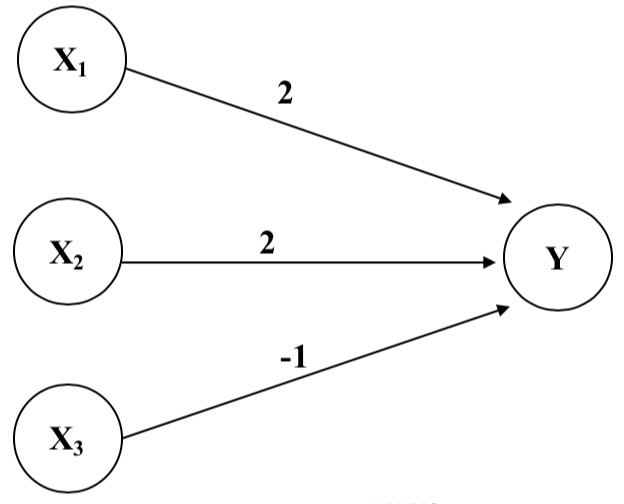• y_in = x1 * 2 + x2 * 2 + x3 * (-1)
• For the network shown here the activation function for unit Y is
• f(y_in) = 1 (fire), if y_in >= θ; else 0 (not fire)
• where y_in is the total input signal received (weighted sum);
• θ is the threshold for Y

### 7.1.3 Neural Networks Architectures

• Three different classes of network architectures
• single-layer feed-forward
• multi-layer feed-forward
• recurrent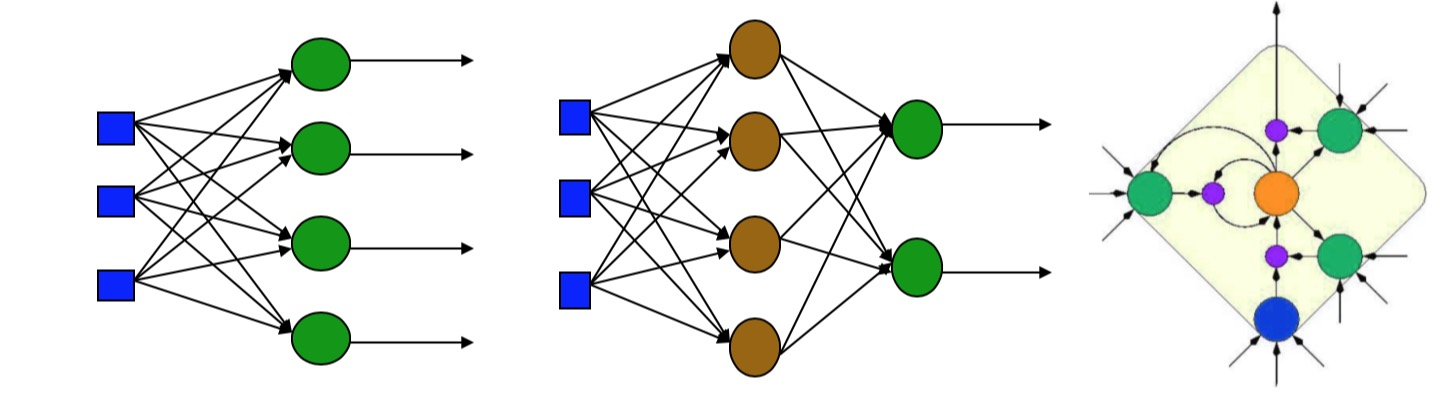### 7.1.4 Linear Separability

• Linearly Separability: classes of patterns with -dimensional vector can be separated with a single decision surface

• Only linearly Separable functions can be represented by a single layer NN (perceptron)

Perceptron: synonym for Single-Layer, Feed-Forward Network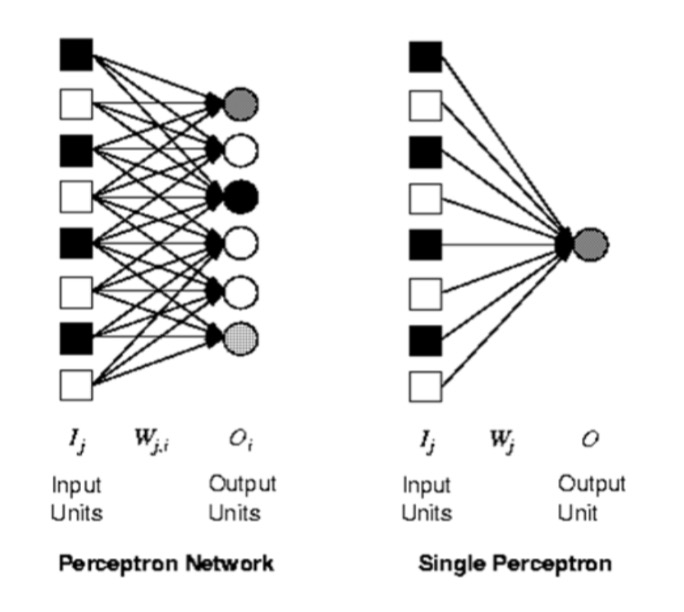### 7.1.5 Training a NN

For an AND Gate: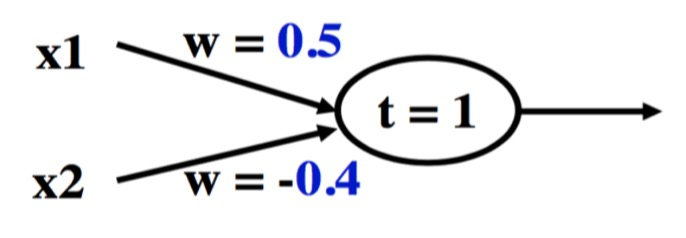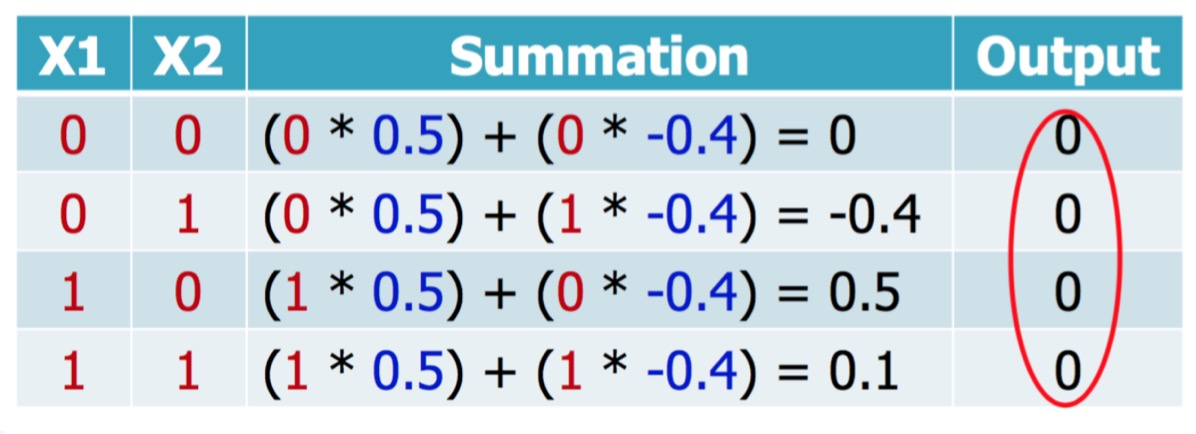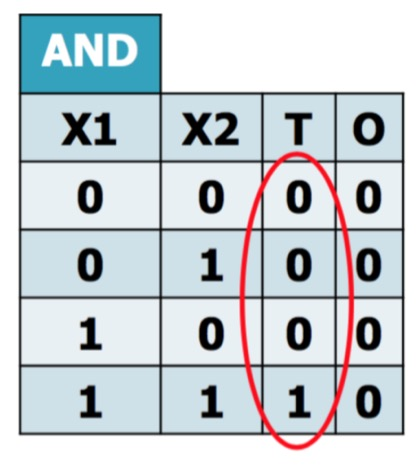• Epoch: the entire training set feed into the neural network
• e.g. The AND function's epoch: ([0,0], [0,1], [1,0], [1,1])
• Training Value (T): the value we require the network to produce
• e.g. the T of [1, 0] is 0
• Error (Err): the amout the output by the network O differs from the training value T
• x_i: inputs to the neuron
• w_i: weight from input x_i to the output
• Learning Rate (LR): how quickly the network converges
• it's set by experimentation, typically 0.1